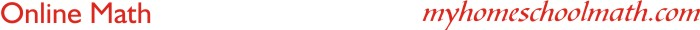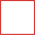Mixed operations - order of operations

 Example 1: Example 2: 35 + 8 x 5 = 35 + (8 x 5) = 35 + 40 = 75                20 ÷ 4 - 2 = (20 ÷ 4) - 2 = 5 - 2 = 3 ==================